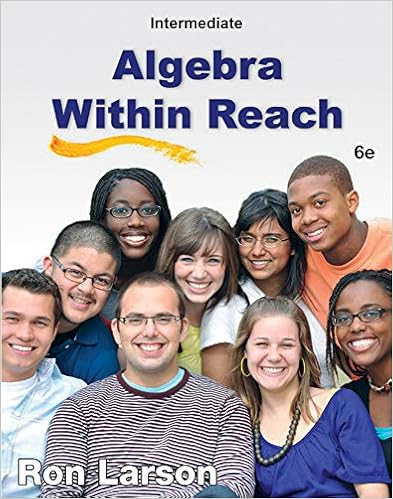# Find the general solution of the system whose

• Homework Help
• 17
• 100% (23) 23 out of 23 people found this document helpful

This preview shows page 5 - 7 out of 17 pages.

##### We have textbook solutions for you!
The document you are viewing contains questions related to this textbook.The document you are viewing contains questions related to this textbook.
Chapter 4 / Exercise 18
Intermediate Algebra Within Reach
LarsonExpert Verified
9.Find the general solution of the system whose augmented matrix is given below.5− 2 2 020− 8 8 015− 6 6 0Choose the correct answer below.A.x1= − 5x2x2= 2x3x3is freeB.x1=25x225x3x2is freex3is freeC.x1= 5x2= − 2x3= 2D.The system has nosolutions.
##### We have textbook solutions for you!
The document you are viewing contains questions related to this textbook.The document you are viewing contains questions related to this textbook.
Chapter 4 / Exercise 18
Intermediate Algebra Within Reach
LarsonExpert Verified
10/23/2016Homework Week 1­Samuel Turner6/1710.In parts(a)through(e)below, mark the statement True or False. Justify each answer.(a)In some cases, a matrix may be row reduced to more than one matrix in reduced echelon form, using differentsequences of row operations.Is this statement true or false?
(b)The row reduction algorithm applies only to augmented matrices for a linear system.Is this statement true or false?
(c)A basic variable in a linear system is a variable that corresponds to a pivot column in the coefficient matrix.Is this statement true or false?
•••